# Expression of a variable from the formula - 7th grade (12y) - math problems

#### Number of problems found: 312

• Temperatures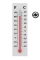Temperatures in degrees Fahrenheit and Celsius are related by the rule F = 1.8C + 32. What is the temperature in degrees Celsius if it is 50°F?
• The expressionWhat is the value of the expression ((62+60))/(23)
• Semicircle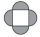The ornament consists of one square and four dark semicircles. The area of the square is 4 cm ^ 2. Find the area of one dark semicircle and round the result to hundreds.
• The product 3The product of the two numbers is 15 ⁵/₆. If one of the numbers is 5 ²/₃, find the other.
• When 5When 5 is subtracted from both my numerator and denominator I become 3/4. What fraction i am?
• A trapezoid 3A trapezoid ABCD has the bases length of a = 120 mm, c = 86 mm and the area A = 2,575 mm2. Find the height of the trapezoid.
• Evaluate expr with 2 varsIf a=6 and x=2, find the value of (2ax + 7x- 10) /(4ax-3a-2)
• A perimeter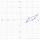A perimeter of a parallelogram is 2.8 meters. The length of one of its sides is equal to one-seventh of the entire perimeter. Find lengths of the sides of the parallelogram.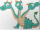Three sides of a quadrilateral are equal to the foreside which is 16 cm long. What is the length of the one with equal sides if the perimeter is 58 cm?
• Equivalent expressionPablo rewrites the expression 8y-24 as 8(y-3). Hana rewrites 8y-24 as 2(4y-12). Are Pablo’s and Hana’s expressions each equivalent to 8y-24? Explain your reasoning.
• A number 5A number is divisible by 24, 25, and 120 if it is increased by 20. Find the number.
• The area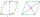The area of a rhombus is 143 m2. If the longer diagonal is 26 m, find the shorter diagonal in inches named d1.
• Original price p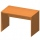If the original price of a table is p and the sales price of the table is p-0.3p, which other expressions represents the sale price of the table? Calculate also for p=100 USD
• A rectangular 3A rectangular plot of land has an area of 3/2 square kilometers and a length of ¾ kilometer.  What is the width of the plot of land?
• Maya is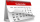Maya is 14 years old. Her brother Jorge is 3 years more than half her age. Which of the following is the correct expression for Jorge's age?
• The perimeter 6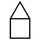The perimeter of quadrilateral is 442.95 cm. If the three known sides measure 84.56 cm,132.77 cm, and 82.75 cm, how long is the fourth side?
• Distributive propertyVerify the distributive property a×(b+c)=(a×b)+(a*c) for the rational number a=5/8, b=7/4 and c=2/3
• Tank B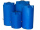Tank B has the same volume as Tank A. The volume of one section of Tank B is 4545 cubic feet. What is the volume, in cubic feet, of the other section of Tank B? Total volume of both tanks A+B is 15000 ft3. Enter your answer in the box.
• The two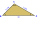The two angles of a triangle are 78° and 82°. So what is the measure of the remaining third angle?
• Two numbers and its productThe product of two numbers are 2/3. If on of them is 1/10, what is the other?

Do you have an exciting math question or word problem that you can't solve? Ask a question or post a math problem, and we can try to solve it.

We will send a solution to your e-mail address. Solved examples are also published here. Please enter the e-mail correctly and check whether you don't have a full mailbox.

Expression of a variable from the formula - math problems. Examples for 7th grade (seventh).